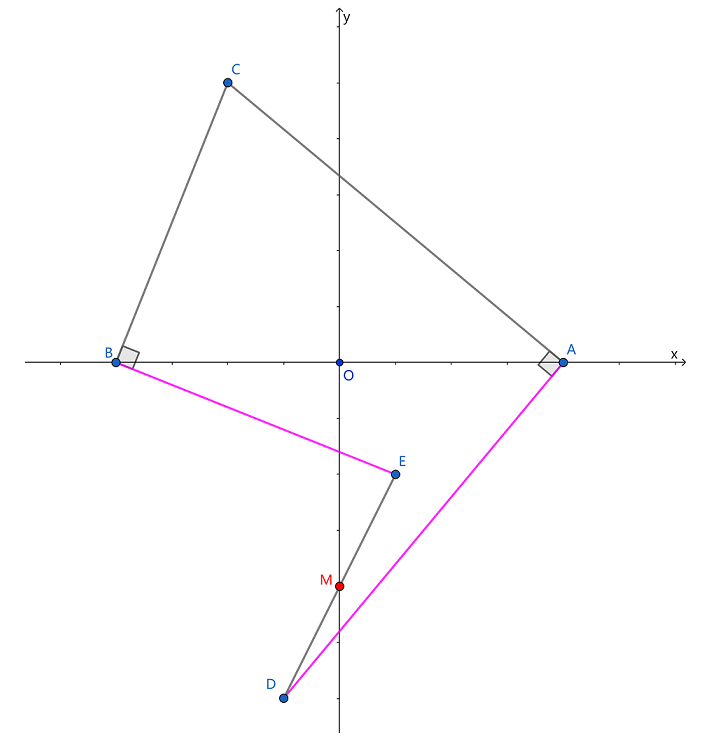安卓手机扫描二维码安装App﻿$\overrightarrow{AC}=z_c-a$﻿

﻿$\overrightarrow{BC}=z_c+a$﻿

﻿$\overrightarrow{AD}=(z_c-a)\mathrm{i}$﻿

﻿$\overrightarrow{BE}=(z_c+a)(-\mathrm{i})$﻿

﻿$DE$﻿ 的中点 ﻿$M$﻿ 对应的复数应为

﻿$k$﻿﻿$[(z_c-a)\mathrm{i}+$﻿﻿$(z_c +a )(- \mathrm{i})]$﻿ ﻿$=-a \mathrm{i}$﻿苹果手机扫描二维码安装App